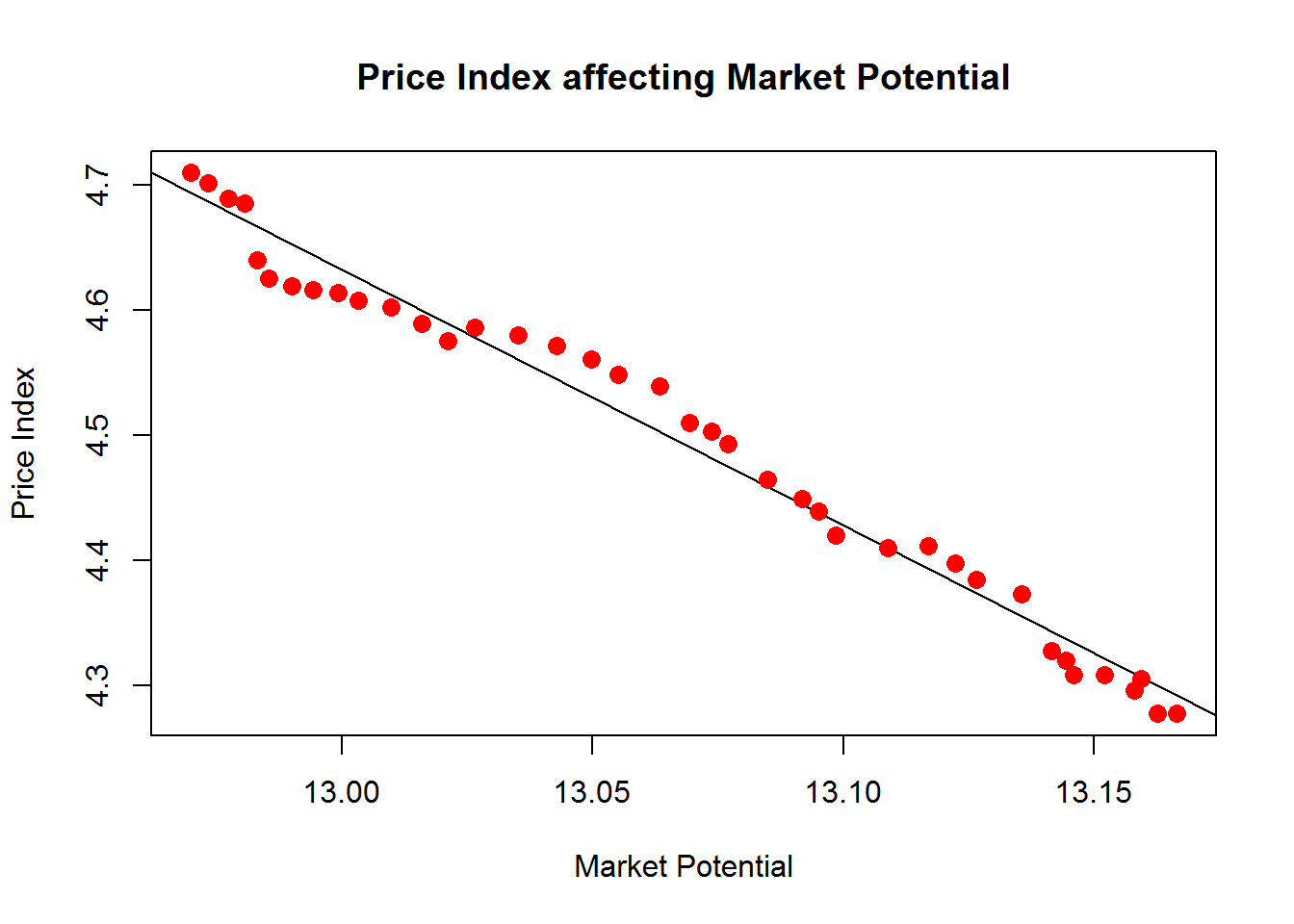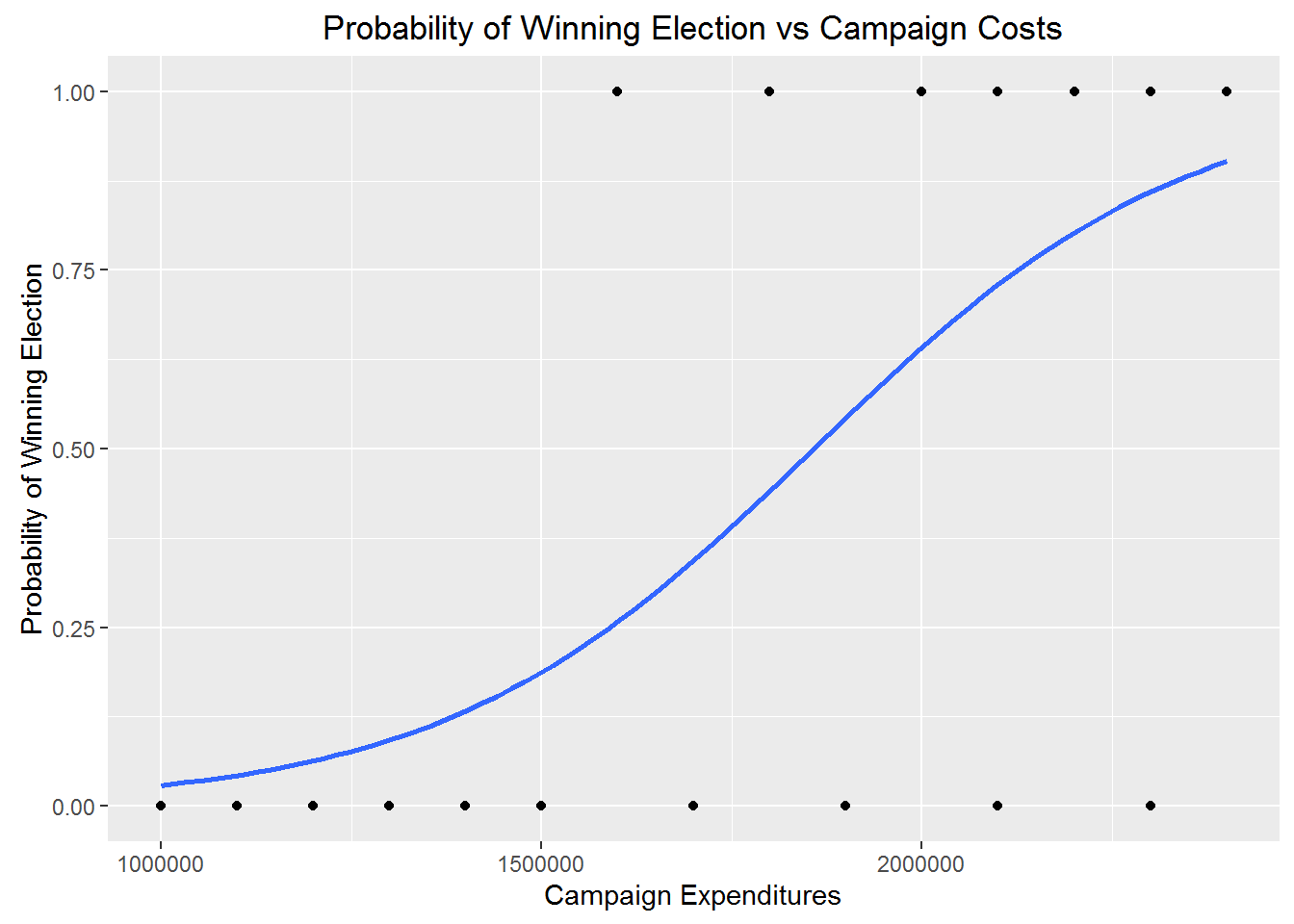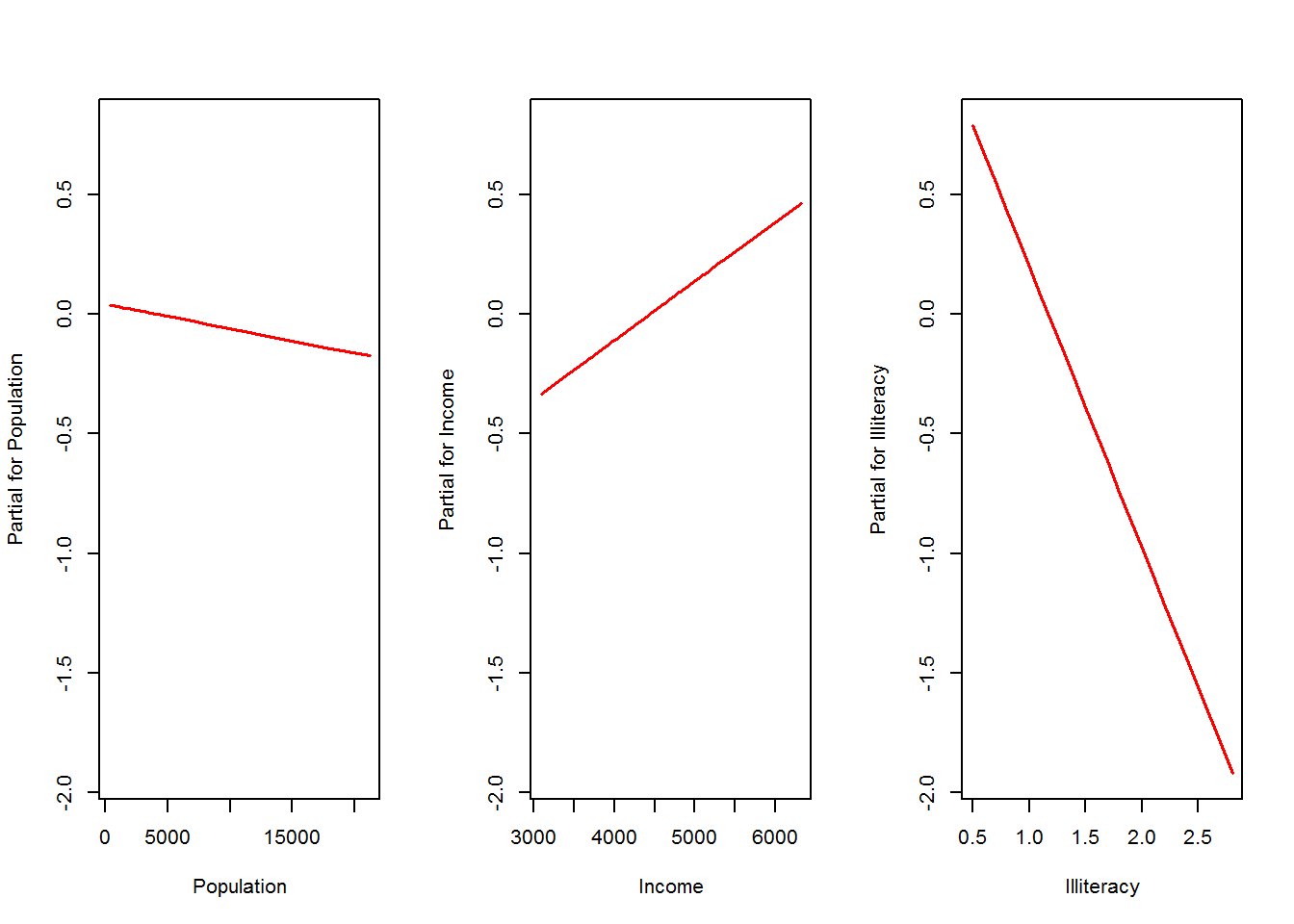## Predictive Analytics Example 1: Linear Regression

Linear Regression allows for prediction of future occurrences derived from one explanatory variable, and one response variable.

library(knitr)
revenue <- freeny.x
kable(head(revenue))
lag quarterly revenue price index income level market potential
8.79636 4.70997 5.82110 12.9699
8.79236 4.70217 5.82558 12.9733
8.79137 4.68944 5.83112 12.9774
8.81486 4.68558 5.84046 12.9806
8.81301 4.64019 5.85036 12.9831
8.90751 4.62553 5.86464 12.9854
colnames(revenue) <- c("lag_quarterly_revenue", "price_index",
"income_level", "market_potential")
revenue <- data.frame(revenue)
model <- lm(market_potential~price_index, revenue)

cat("The Intercept =", model$coefficients) ## The Intercept = 15.21788 test <- data.frame(price_index=4.57592) result <- predict(model, test) plot(revenue$market_potential, revenue$price_index, col = "red", main = "Price Index affecting Market Potential", abline(lm(revenue$price_index~revenue$market_potential)), cex = 1.3, pch = 16, xlab = "Market Potential", ylab = "Price Index")## Example 1 - Linear Regression Conclusion: cat("For a Price Index of ", as.character(test), ", the predicted Market Potential = ", round(result, 2), ".", sep="") ## For a Price Index of 4.57592, the predicted Market Potential = 13.03. ### In conclusion to ContextBase Predictive Analytics Example 1, a direct correlation of Price Index to Market Potential was found, (see above graph). As a test of the Predictive Algorithm, a Price Index of 4.57592 was processed, and a Market Potential of 13.03 was predicted. The source R dataset shows this prediction to be accurate. ## Predictive Analytics Example 2: Logistic Regression Logistic Regression allows for prediction of a logical, (Yes or No), occurrence based on the effects of an explanatory variable on a response variable. For example, the probability of winning a congressional election vs campaign expenditures. How does the amount of money spent on a campaign affect the probability that the candidate will win the election? Expenditures <- c(1000000, 1100000, 1200000, 1300000, 1400000, 1500000, 1600000, 1700000, 1800000, 1900000, 2000000, 2100000, 2200000, 2300000, 2400000, 2000000, 2100000, 2200000, 2300000, 2400000) ElectionResult <- c(0, 0, 0, 0, 0, 0, 1, 0, 1, 0, 1, 0, 1, 0, 1, 1, 1, 1, 1, 1) CampaignCosts <- data.frame(Expenditures, ElectionResult) kable(CampaignCosts, caption = "Campaign Expenditures of Candidates vs Election Results") Campaign Expenditures of Candidates vs Election Results Expenditures ElectionResult 1000000 0 1100000 0 1200000 0 1300000 0 1400000 0 1500000 0 1600000 1 1700000 0 1800000 1 1900000 0 2000000 1 2100000 0 2200000 1 2300000 0 2400000 1 2000000 1 2100000 1 2200000 1 2300000 1 2400000 1 library(ggplot2) ggplot(CampaignCosts, aes(Expenditures, ElectionResult)) + geom_point(aes()) + geom_smooth(method='glm', method.args = list(family = "binomial"), se=FALSE) + labs (x="Campaign Expenditures", y="Probability of Winning Election", title="Probability of Winning Election vs Campaign Costs")The logistic regression analysis gives the following output: model <- glm(ElectionResult ~., family=binomial(link='logit'), data=CampaignCosts) model$coefficients
##   (Intercept)  Expenditures
## -7.615054e+00  4.098080e-06

The output indicates that campaign expenditures significantly affect the probability of winning the election. The output provides the coefficients for Intercept = -7.615054e+00, and Expenditures = 4.098080e-06. These coefficients are entered in the logistic regression equation to estimate the probability of winning the election:

Probability of winning election = 1/(1+exp(-(-7.615054e+00+4.098080e-06*CampaignExpenses)))

For a Candidate that has $1,600,000 in expenditures: CampaignExpenses <- 1600000 ProbabilityOfWinningElection <- 1/(1+exp(-(-7.615054e+00+4.098080e-06*CampaignExpenses))) cat("Probability of winning Election = 1/(1+exp(-(-7.615054e+00+4.098080e-06*", CampaignExpenses, "))) = ", round(ProbabilityOfWinningElection, 2), ".", sep="") ## Probability of winning Election = 1/(1+exp(-(-7.615054e+00+4.098080e-06*1600000))) = 0.26. For a Candidate that has$2,100,000 in expenditures:

CampaignExpenses <- 2100000
ProbabilityOfWinningElection <- 1/(1+exp(-(-7.615054e+00+4.098080e-06*CampaignExpenses)))

cat("Probability of winning Election = 1/(1+exp(-(-7.615054e+00+4.098080e-06*",
CampaignExpenses, "))) = ", round(ProbabilityOfWinningElection, 2), ".", sep="")
## Probability of winning Election = 1/(1+exp(-(-7.615054e+00+4.098080e-06*2100000))) = 0.73.

## Example 2 - Logistic Regression Conclusion:

ElectionWinTable <- data.frame(column1=c(1100000, 1400000,
1700000, 1900000,
2300000),
column2=
c(round(1/(1+exp(-(-7.615054e+00+4.098080e-06*1100000))), 2),
round(1/(1+exp(-(-7.615054e+00+4.098080e-06*1400000))), 2),
round(1/(1+exp(-(-7.615054e+00+4.098080e-06*1700000))), 2),
round(1/(1+exp(-(-7.615054e+00+4.098080e-06*1900000))), 2),
round(1/(1+exp(-(-7.615054e+00+4.098080e-06*2300000))), 2)))
names(ElectionWinTable) <- c("Campaign Expenses", "Probability of Winning Election")
kable(ElectionWinTable)
Campaign Expenses Probability of Winning Election
1100000 0.04
1400000 0.13
1700000 0.34
1900000 0.54
2300000 0.86

## Predictive Analytics Example 3: Multiple Regression

Multiple Regression allows for the prediction of the future values of a response variable, based on values of multiple explanatory variables.

input <- data.frame(state.x77[,1:4])
kable(head(input))
Population Income Illiteracy Life.Exp
Alabama 3615 3624 2.1 69.05
Arizona 2212 4530 1.8 70.55
Arkansas 2110 3378 1.9 70.66
California 21198 5114 1.1 71.71
colnames(input) <- c("Population", "Income", "Illiteracy", "Life_Exp")

# Create the relationship model.
model <- lm(Life_Exp~Population+Income+Illiteracy, data=input)

# Show the model.
print(model)
##
## Call:
## lm(formula = Life_Exp ~ Population + Income + Illiteracy, data = input)
##
## Coefficients:
## (Intercept)   Population       Income   Illiteracy
##   7.120e+01   -1.024e-05    2.477e-04   -1.179e+00
# Multiple Regression Plot
par(mfrow=c(1,3))
termplot(model)a <- coef(model)
cat("The Multiple Regression Intercept = ", a, ".", sep="")
## The Multiple Regression Intercept = 71.2023.
XPopulation <- coef(model)
XIncome <- coef(model)
XIlliteracy <- coef(model)

modelCoef <- data.frame(XPopulation, XIncome, XIlliteracy)
colnames(modelCoef) <- c("Population", "Income", "Illiteracy")
row.names(modelCoef) <- c("Coefficients")
kable(modelCoef)
Population Income Illiteracy
Coefficients -1.02e-05 0.0002477 -1.178788

## Multiple Regression Conclusion:

popl <- 3100
Incm <- 5348
Illt <- 1.1

Y = a + popl * XPopulation + Incm * XIncome + Illt * XIlliteracy
cat("For a City where Population = ", popl, ", Income = ", Incm,  ", and Illiteracy = ", Illt, ",
the predicted Life Expectancy is: ", round(Y, 2), ".", sep="")
## For a City where Population = 3100, Income = 5348, and Illiteracy = 1.1,
## the predicted Life Expectancy is: 71.2.